# AP Physics C: Electricity and Magnetism Practice Test 3

### Test Information10 questions13 minutes

1.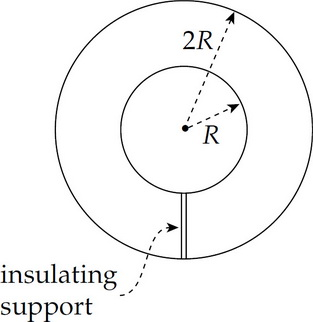The figure above shows a cross section of two concentric spherical metal shells of radii R and 2R, respectively. Find the capacitance.

2. Traveling at an initial speed of 1.5 × 106 m/s, a proton enters a region of constant magnetic field, B, of magnitude 1.0 T. If the proton's initial velocity vector makes an angle of 30° with the direction of B, compute the proton's speed 4 s after entering the magnetic field.

3. There is initially no current through any circuit element in the following diagram.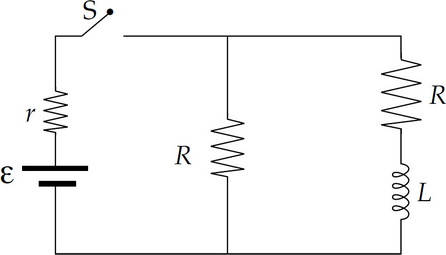What is the current through r immediately after the switch S is closed?

4. There is initially no current through any circuit element in the following diagram.After the switch has been kept closed for a long time, how much energy is stored in the inductor?

5. There is initially no current through any circuit element in the following diagram.After having been closed for a long time, the switch is suddenly opened. What is the current through r immediately after S is opened?

6.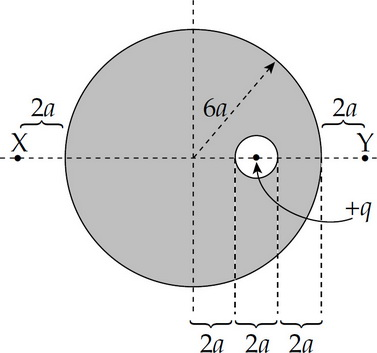A solid, neutral metal sphere of radius 6a contains a small cavity, a spherical hole of radius a as shown above. Within this cavity is a charge, +q. If EX and EY denote the strength of the electric field at points X and Y respectively, which of the following is true?

7.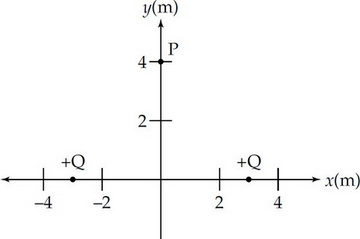Two particles of charge +Q are located on the x-axis, as shown above. Determine the work done by the electric field to move a particle of charge –Q from very far away to point P.

8. A battery is connected in series with a switch, a resistor of resistance R, and an inductor of inductance L. Initially, there is no current in the circuit. Once the switch is closed and the circuit is completed, how long will it take for the current to reach 99% of its maximum value?

9. What is the maximum number of 40 W light bulbs that could be connected in parallel with a 120 V source? The total current cannot exceed 5 A or the circuit will blow a fuse.

10.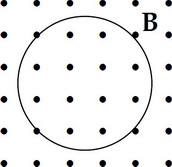The metal loop of wire shown above is situated in a magnetic field B pointing out of the plane of the page. If B decreases uniformly in strength, the induced electric current within the loop is